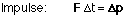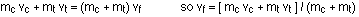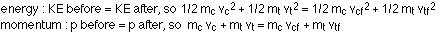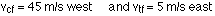## Momentum

10-20-99

Sections 7.1 - 7.5

### Momentum

There are two kinds of momentum, linear and angular. A spinning object has angular momentum; an object traveling with a velocity has linear momentum. For now, and throughout chapter 7, we'll deal with linear momentum, and just refer to it as momentum, without the linear.

There are 4 really important things to know about momentum. The first is how momentum is defined, as the product of mass times velocity:

momentum : p = mv

The second note is built into this equation; momentum is a vector, and the momentum has the same direction as the velocity.

The third point is the relationship between momentum and force. We've talked a lot about forces in the last few weeks, and there is a strong connection between force and momentum. In fact, Newton's second law was first written (by Newton himself, of course) in terms of momentum, rather than acceleration. A force acting for a certain time (this is known as an impulse) produces a change in momentum.Again, this is a vector equation, so the change in momentum is in the same direction as the force.

The fourth really important point about momentum is that momentum is conserved; the total momentum of an isolated system is constant. Note that "isolated" means that no external force acts on the system, which is a set of interacting objects. If a system does have a net force acting, then the momentum changes according to the impulse equation.

Momentum conservation applies to a single object, but it's a lot more interesting to look at a situation with at least two interacting objects. If two objects (a car and a truck, for example) collide, momentum will always be conserved. There are three different kinds of collisions, however, elastic, inelastic, and completely inelastic. Just to restate, momentum is conserved in all three kinds of collisions. What distinguishes the collisions is what happens to the kinetic energy.

Types of collisions: (momentum is conserved in each case)

• elastic - kinetic energy is conserved
• inelastic - kinetic energy is not conserved
• completely inelastic - kinetic energy is not conserved, and the colliding objects stick together after the collision.

The total energy is always conserved, but the kinetic energy does not have to be; kinetic energy is often transformed to heat or sound during a collision.

### A1-D collision example

A car of mass 1000 kg travels east at 30 m/s, and collides with a 3000 kg truck traveling west at 20 m/s.
(a) If the collision is completely inelastic, how fast are the car and truck going, and in what direction, after the collision? What percentage of the kinetic energy is lost in the collision?
(b) What happens if the collision is elastic?

(a) Car crashes are often completely inelastic, with much of the kinetic energy going into deforming the cars. Momentum is always conserved, though, so, using c for car and t for truck, (and f for final) the conservation of momentum equation is:If we take east as the positive direction, then the truck's velocity goes into the equation with a negative sign, so: vf = [ (1000) (30) + (3000) (-20) ] / (1000 + 3000) = -7.5 m/s, which is 7.5 m/s west

The change in kinetic energy can be found by adding up the kinetic energy before and after the collision:KE lost = 1050000 - 112500 = 937500 J
Percentage of KE lost = 100% x 937500 / 1050000 = 89.3%

So, a great deal of the kinetic energy is lost in the collision.

(b) What would happen if the car and truck were both made out of rubber and the collision was elastic, with no loss of kinetic energy. In this case the calculations are a lot more complicated, because we have to combine the energy conservation equation with the momentum conservation equation:In this case, after some nice algebraic manipulation (which is worth trying on your own), the final velocities of the car and truck work out to:Note that if you were driving the car, you would experience a much greater force in the case of an elastic collision than in a completely inelastic collision, in which much of the energy is absorbed by the deformation of the car. Let's say you have a mass of 50 kg, and that the collision lasts for 0.1 seconds. In the case of the completely inelastic collision, your momentum would change from 50 kg x 30 m/s east = 1500 kg m/s east to 50 kg x 7.5 m/s west = -375 kg m/s east, which is a net change of 1875 kg m/s. This change in momentum is produced by an average force acting for the 0.1 s of the collision, so the force works out to 18750 N.

In the elastic collision, your momentum would change from 1500 kg m/s east to 50 kg x 45 m/s west = -2250 kg m/s east, for a net change of 3750 kg m/s, exactly twice that in the completely inelastic case. The force you would experience would therefore also be doubled.

### Back to impulse

Before doing an example of a collision in 2 dimensions, let's look at a short example of how the impulse equation is applied. Recall that impulse is a force acting for a particular time, producing a change in momentum:Consider a hose spraying water directly at a wall. If 3 kg of water emerge from the hose every second, and the speed of the water is 10 m/s, how much force is exerted on the wall by the water?

The first step in coming to an answer is making an assumption, that the water does not bounce back from the wall, but is simply stopped by the wall. In this case, the change in momentum for one second's worth of water is -30 kg m/s. To produce this change in momentum, the wall must exert a force on the water of -30 N, which is 30 N in the direction opposite to the direction the water travels from the hose. The water exerts an equal and opposite force on the wall, 30 N in the direction the hose points.

Note that is the water bounced off the wall and came back with a momentum of 30 kg m/s towards the hose, that would represent a net change in momentum of 60 kg m/s towards the hose, because momentum is a vector. In that case the force exerted by the water on the wall would be twice as high, 60 N.